• 这是一个MWE： from pyspark.sql.types import IntegerType from pyspark.sql.functions import explode, col, udf, mean as _mean, stddev as _stddev # mock up sample dataframe df = sqlCtx.createDataFrame( ...
您可以使用内置函数来获取聚合统计信息。以下是如何获得均值和标准偏差。from pyspark.sql.functions import mean as _mean, stddev as _stddev, coldf_stats = df.select(_mean(col('columnName')).alias('mean'),_stddev(col('columnName')).alias('std')).collect()mean = df_stats['mean']std = df_stats['std']请注意，有三种不同的标准偏差功能。从文档中我使用的文档(stddev)返回以下内容：聚合函数：返回无偏样本标准差组中的表达您也可以使用describe()方法：df.describe().show()更新：这是您处理嵌套数据的方法。使用explode将值提取到单独的行中，然后调用mean和stddev，如上所示。这是一个MWE：from pyspark.sql.types import IntegerTypefrom pyspark.sql.functions import explode, col, udf, mean as _mean, stddev as _stddev# mock up sample dataframedf = sqlCtx.createDataFrame([(680, [[691,1], [692,5]]), (685, [[691,2], [692,2]]), (684, [[691,1], [692,3]])],["product_PK", "products"])# udf to get the "score" value - returns the item at index 1get_score = udf(lambda x: x, IntegerType())# explode column and get statsdf_stats = df.withColumn('exploded', explode(col('products')))\.withColumn('score', get_score(col('exploded')))\.select(_mean(col('score')).alias('mean'),_stddev(col('score')).alias('std'))\.collect()mean = df_stats['mean']std = df_stats['std']print([mean, std])哪个输出：[2.3333333333333335, 1.505545305418162]您可以使用numpy验证这些值是否正确：vals = [1,5,2,2,1,3]print([np.mean(vals), np.std(vals, ddof=1)])说明：您的"products"列是list的{​​{1}}。调用list将为外部explode的每个元素创建一个新行。然后从每个展开的行中获取list值，这些行已定义为2元素"score"中的第二个元素。最后，在这个新列上调用聚合函数。
展开全文• .reduce(_ + _) .divide(df.columns.size - 1) .alias("mean")) 在更复杂的场景中，可以使用 array函 array合列，并使用UDF计算统计信息： import numpy as np from pyspark.sql.functions import array, udf from ...
这里只需要一个这样的标准SQL：SELECT (US + UK + CAN) / 3 AS mean FROM df可以直接与sqlcontext.sql一起使用，也可以使用dsl表示df.select(((col("UK") + col("US") + col("CAN")) / lit(3)).alias("mean"))如果有更多的列，可以按如下方式生成表达式：from functools import reducefrom operator import addfrom pyspark.sql.functions import col, litn = lit(len(df.columns) - 1.0)rowMean  = (reduce(add, (col(x) for x in df.columns[1:])) / n).alias("mean")df.select(rowMean)或rowMean  = (sum(col(x) for x in df.columns[1:]) / n).alias("mean")df.select(rowMean)最后，它在scala中的 equivalent是：df.select(df.columns.drop(1).map(col).reduce(_ + _).divide(df.columns.size - 1).alias("mean"))在更复杂的场景中，可以使用 array函 array合列，并使用UDF计算统计信息：import numpy as npfrom pyspark.sql.functions import array, udffrom pyspark.sql.types import FloatTypecombined = array(*(col(x) for x in df.columns[1:]))median_udf = udf(lambda xs: float(np.median(xs)), FloatType())df.select(median_udf(combined).alias("median"))使用scala api表示的相同操作：val combined = array(df.columns.drop(1).map(col).map(_.cast(DoubleType)): _*)val median_udf = udf((xs: Seq[Double]) =>breeze.stats.DescriptiveStats.percentile(xs, 0.5))df.select(median_udf(combined).alias("median"))由于spark 2.4，另一种方法是将值组合到 array中并应用聚合表达式。例如，请参阅Spark Scala Row-Wise Average by Handling null。
展开全文• df = pandas.read_excel('test.xlsx') df = pandas.pivot_table(df, index=['a'], columns=['b'], aggfunc=numpy.sum) ...想计算周日均数据变成这样 pv uv 20180105~20180111 1 1 20180111~20180118 2 2python
• 小编做为一个多年资深搞仓库卫生，管理库存计划的打工人，由于工作需要，也接触了几种不同的计算平均值的方法，虽然现在系统是越来越强大了，很多数据，系统都能够直接计算出来的。但是，知其然才能知其所以然，了解...
小编做为一个多年资深搞仓库卫生，管理库存计划的打工人，由于工作需要，也接触了几种不同的计算平均值的方法，虽然现在系统是越来越强大了，很多数据，系统都能够直接计算出来的。但是，知其然才能知其所以然，了解下计算的原理，掌握最基本的原则还是很重要的。只有这样，我们才能更好的开展工作。今天，我们就以配件预算月需求这个实例来看看，几种不同的计算平均值方法，用excel表格，需要怎么计算。1、计算算数平均值科普下，一般对于使用有1年或以上的配件，默认是以前12个月的平均值来预测这个很简单了，就是12个月的数量相加，然后除于12个月求平均值，如果不用公式，可以如图这样。恩恩，硬核……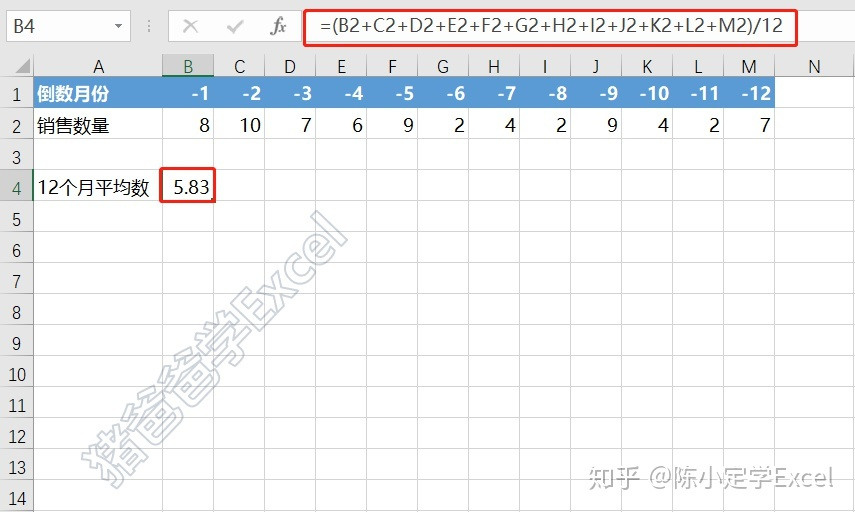好了，不开玩笑，一个average函数能搞定的事，就不搞笑了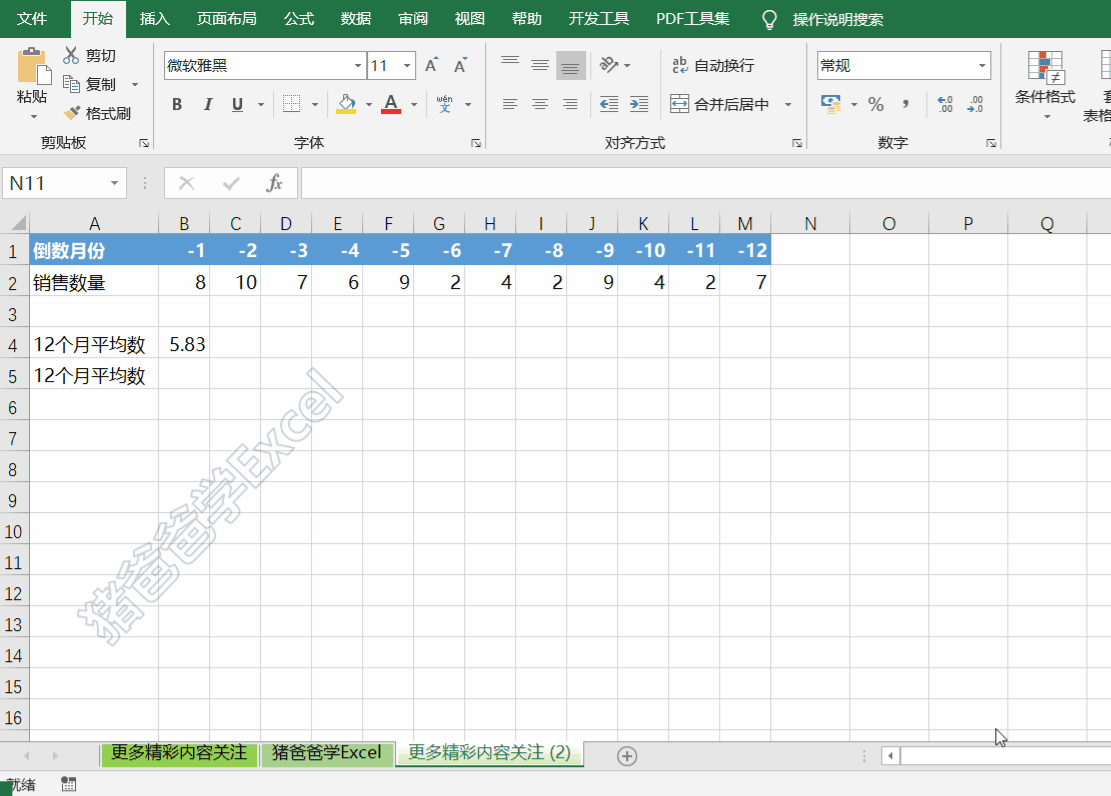2、计算加权平均值而对有半年以上而还未满一年的配件，一般我们就使用加权平均值来进行预测。概念：加权平均值即将各数值乘以相应的权数，然后加总求和得到总体值，再除以总的单位数。如下图所示。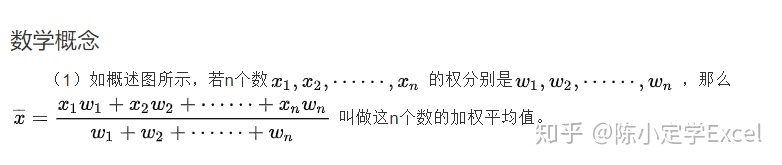那么，我们就可以使用之前也有讲过sumproduct函数和sum函数来进行计算。相应公式就是类似=SUMPRODUCT(C2:H2,$C$7:$H$7)/SUM(C7:H7)，而又因为这里的各个数据的总权重为1，所以就可以将UM(C7:H7)省略，最终公式就是直接使用sumproduct即可。如动图所示，在O2单元输入公式=SUMPRODUCT(C2:H2,$C$7:$H$7)，注意权重部分的$C$7:$H$7需要使用绝对引用，这样在复制公式时，才不会因为权重部分引用错误而导致计算错误。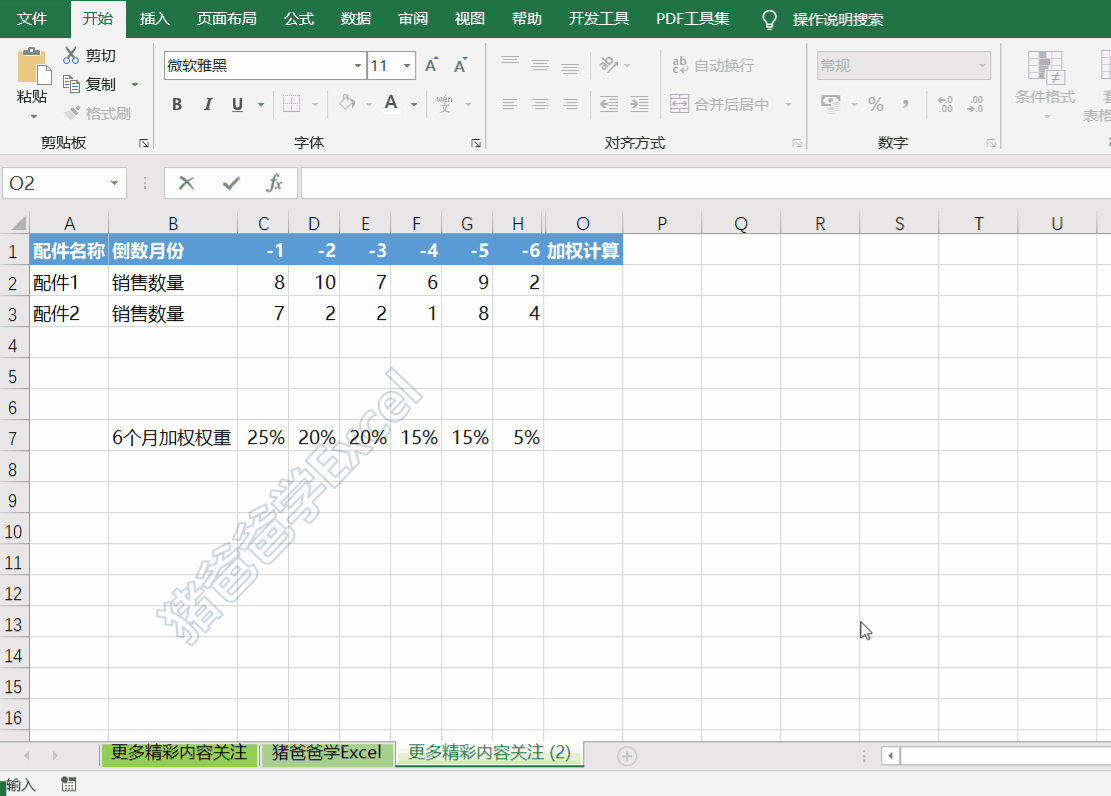有关sumproduct函数，如果有不明白的，可以看回这篇文章了解sumproduct函数——哪里需要去哪里，求和计数样样精通​mp.weixin.qq.com当然，配件预测销售数量一般不可能有小数点，这里，再设置多一个取整函数就可以了，就不展开了。可以看这篇眼见不一定为实，四舍五入取整函数来帮忙​mp.weixin.qq.com3、根据指定条件求平均值如动图，我们要计算总经办的平均工资，这里的条件，就是部门为总经办。我们来使用一个比average功能强大一点的兄弟，averageifs函数，先来了解下语法。作用：返回满足多个条件的所有单元格的平均值（算术平均值）。AVERAGEIFS(average_range,criteria_range1, criteria1, [criteria_range2, criteria2], ...)AVERAGEIFS函数语法具有下列参数：Average_range    必需。要计算平均值的一个或多个单元格，其中包含数字或包含数字的名称、数组或引用。Criteria_range1、criteria_range2等   Criteria_range1 是必需的，后续 criteria_range 是可选的。在其中计算关联条件的 1 至 127 个区域。Criteria1、criteria2等    Criteria1 是必需的，后续 criteria 是可选的。形式为数字、表达式、单元格引用或文本的 1 至 127 个条件，用来定义将计算平均值的单元格。例如，条件可以表示为 32、"32"、">32"、"苹果" 或 B4。语法这么长，其实简单理解，就是AVERAGEIFS(求平均值区域，条件区域1，条件1，……),可以了。在此例，我们在G3单元格输入公式=AVERAGEIFS(C3:C12,A3:A12,F3)，这里求平均值的区域，就是C3:C12；而条件区域就是A3:A12，相对应条件就是F3单元格的“总经办”。这样，我们的总经办平均工资就求出来了。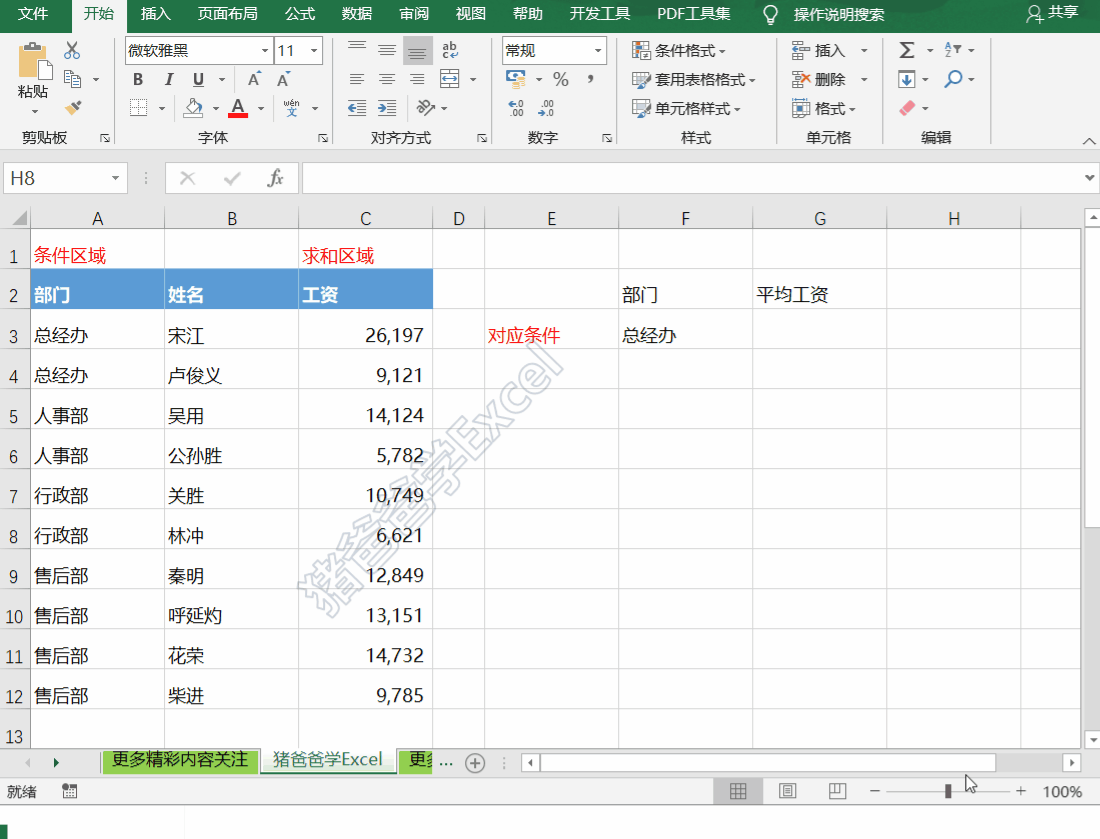大脑：恩，看懂了~手：你说啥？鼠标要点哪里？要怎么点击？光看不练，上阵头晕，大家多多练习下，才能真正掌握哦~~今天的分享就到这里，大家生活愉快~~赞同、喜欢、收藏~~~都可以有的~~向我砸过来哈~~
展开全文• 上节介绍获取行、列子集的方法，运用了range函数和python的切片语法，在数据分析中，总是会进行统计计算，很多基本统计计算有现成的库可用，或内置在语言中。下面以data数据可以先问下面几个问题。1.数据中每年的...


上节介绍获取行、列子集的方法，运用了range函数和python的切片语法，在数据分析中，总是会进行统计计算，很多基本统计计算有现成的库可用，或内置在语言中。下面以data数据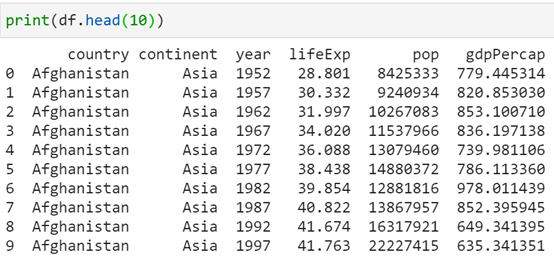可以先问下面几个问题。1.     数据中每年的平均预期寿命是多少？平均预期寿命、人口和GDP是多少？2.     如果按洲对数据分层，并进行同样的计算，结果会怎样？3.     每个洲列出了多少个国家和地区？对于上面的问题，需要进行分组计算(即聚合)。换句话说，需要进行一个计算，可以是平均计算或者频率计算，并将其应用于变量的每个子集。可以把分组计算看作“分割-应用-组合”的过程，首先把数据分割成若干部分，然后把选择的函数(或计算)应用于各部分，最后把所有独立的分割计算组合成一个Data Frame。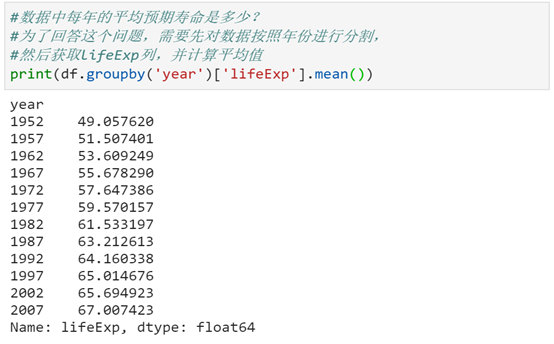拆解上面的语句，首先创建一个分组对象。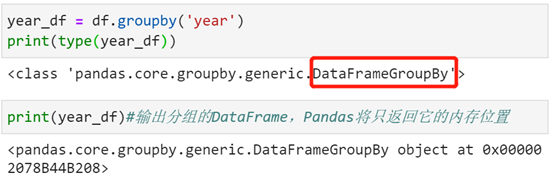可以根据分组数据中选取需要计算的列，对它们进行计算。要回答上面的问题，需要获取lifeExp列。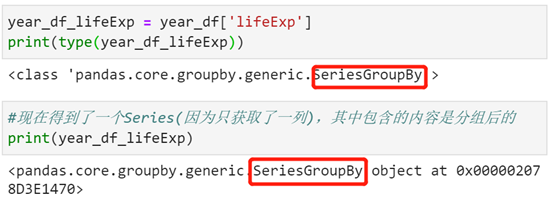最后计算数字向量的平均值，就得到了最终的期望结果。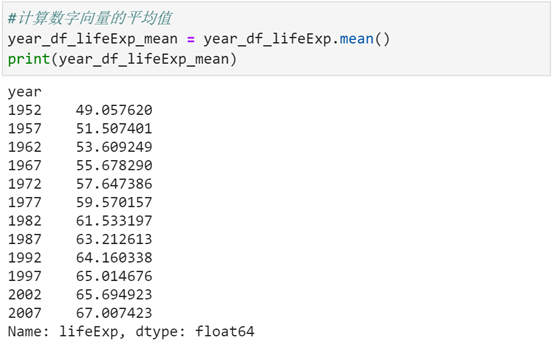可以对人口和GDP做一系列类似的计算，如果想使用多个变量对数据进行分组和分层，应该怎么办呢？想对多列执行相同的计算呢？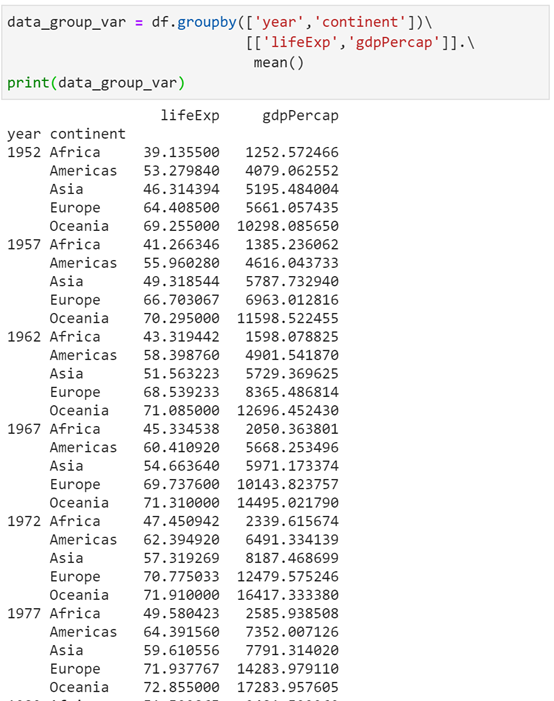上面输出的数据是按年份和洲进行分组，针对每个一个“年份-洲”对，计算平均预期寿命和平均GDP。输出的数据有点奇怪。如果想平铺Data Frame，使用reset_index方法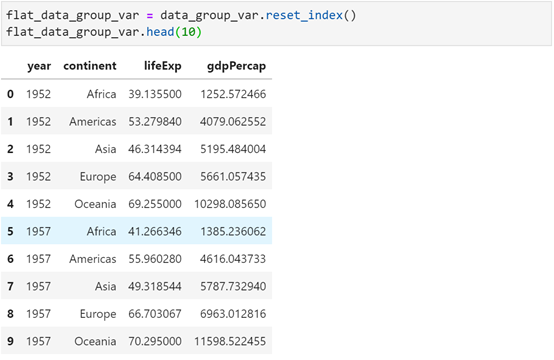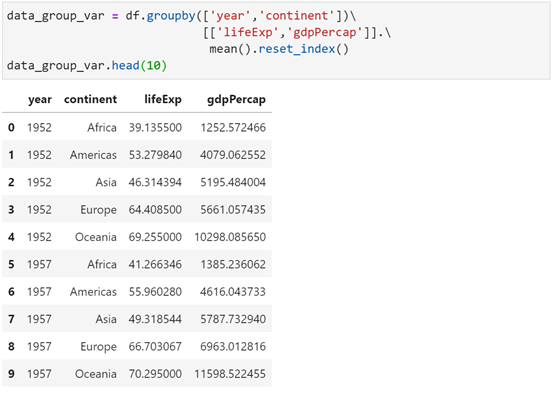数据相关的另一个常见任务是计算频率。可使用numique方法或value_counts方法获取Pandas Series的唯一值计数或频率计数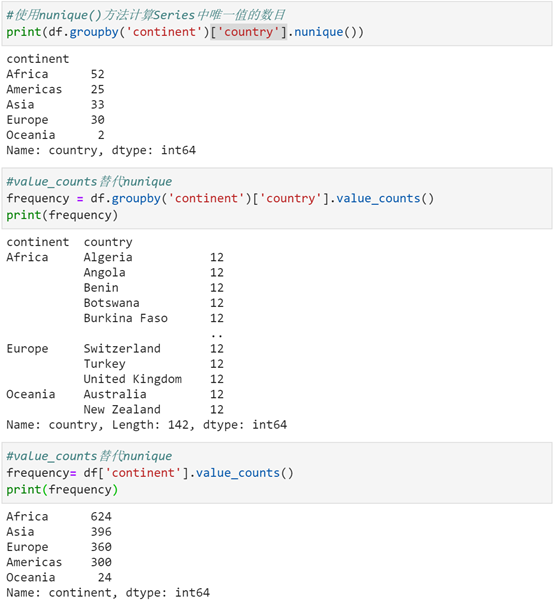可视化在数据处理的每个步骤中都非常重要。在理解和清理数据时，可视化有助于识别数据中的趋势，并展示最终的发现，使用pandas创建一些基本图，下面人口各年底的预期寿命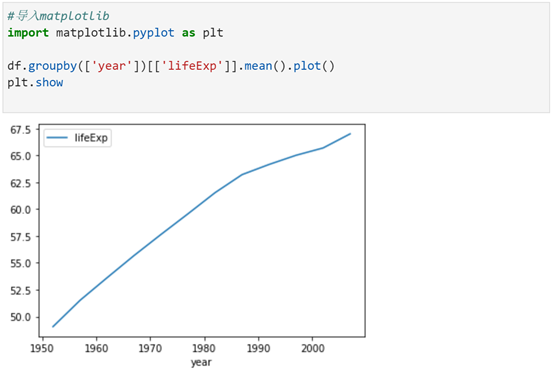Dara Frame基础知识就全部完了，即将入门下一个模块。资料链接：https://pan.baidu.com/s/18veIg3cFCDEooKbqRq4w_w提取码：0010数据分析Pandas运用 | DataFrame基础知识.(7-3)数据分析Pandas运用 | DataFrame基础知识.(7-2)数据分析Pandas运用 |  DataFrame基础知识.(7-1)

展开全文• pandas中Series和DataFrame基本操作。设有DataFrame结果的数据a如下所示：a bcone4 1 1two6 2 0three6 1 6一、查看数据(查看对象的方法对于Series来说同样适用)1.查看DataFrame前xx行或后xx行a=DataFrame(data);a....
• 16_Pandas.DataFrame计算统计信息并按GroupBy分组 ...计算平均值，最小值，最大值，总和等 通过应用任意处理进行聚合：agg（） 批量统计关键统计信息：describe（） 绘制图表 iris数据集 以iris数据集为python 机器学习
• 如何查看Pandas DataFrame对象列的最大值、最小值、平均值、标准差、中位数等 我们举个例子说明一下，先创建一个dataframe对象df，内容如下: 1.使用sum函数获得函数列的和,用法:df.sum() 2.使用max获取最大值,用法...
• 首先把你的数据放到panda数据框中——我自己编了一个虚拟...启动框架import pandas as pdimport datetimedf1 = pd.DataFrame({'Start_date' : ['2018-01-01','2018-01-02','2018-01-03','2018-02-01','2018-03-10...
• Python中如何求DataFrame中某一列数的日平均值？ 例如求df对象中close列的5日均线： 1.使用循环，采用手动计算的方式： df['close'][i-4:i+1].mean() 获取第i天的5日均线 2.对整个dataframe对象，使用rolling...
• 作者：彭佳 转自： Excel之家ExcelHome小伙伴们好啊，今天和大家分享一组用于平均值计算的函数，分别...是要计算平均值的 1～255 个数值或是单元格区域。如果单元格引用参数中包含文字、逻辑值或空单元格，则忽略这...
• 首先通过a和b列定义的范围创建范围DataFrame：a = np.arange(0, 180, 30)df1 = pd.DataFrame({'aa':a[:-1], 'bb':a[1:]})#print (df1)然后通过helper列tmp交叉连接所有行：^{pr2}\$最后一个过滤器-有两个按列的解决...
• 有没有一种简单的方法来计算pandas DataFrame中每一列的平均值,并且为每一行排除特定值？下面每一行中的x标记了每次迭代中要从计算中排除的值：a b a b a b0 1 2 0 x x 0 1 21 ...
• 本人今天遇到遇到一个任务，计算同月份合约当天各合约总持仓量的移动平均值。立刻写下了这个函数： group = df.groupby(['合约系列','date']) f = pd.DataFrame(group['持仓量'].sum().rolling(20).mean()) 上交...python
• 数据聚合与分组运算——GroupBy技术(1)，有需要的朋友可以参考下。...计算分组摘要统计，如计数、平均值、标准差，或用户自定义函数。对DataFrame的列应用各种各样的函数。应用组内转换或其他运算，如规格...
• i）添加用于存放各索引分组平均值的列假设我们想要为一个DataFrame添加一个用于存放各索引分组平均值的列。一个办法是先聚合再合并：先聚合：再合并：虽然这样也行，但是不太灵活。你可以将该过程看作利用np.me...
• 数据分组分组统计 - groupby功能① 根据某些条件将数据拆分成组② 对每个组独立应用函数③ 将结果合并到一个数据结构中Dataframe在行(axis=0)或列(axis=1)上进行分组，将一个函数应用到各个分组并产生一个新，然后...
• 前言上一篇（戳我查看）Pandas的语句介绍到DataFrame的滑片，这篇就继续介绍Pandas中的一些基本语句。数据清洗df.columns = ['a','b','c'] # 重命名列名称 pd.isnull() # 检查是否为null，为nul...
• 回顾 在数据处理进阶pandas入门(十六)中，我们介绍了pandas中分组方法groupby()的各种分组方式以及多计算方法agg()的基本概念与用法。今天我们讲一下对groupby()方法分组数据处理的两个方法：数据分组转换transform...
• I have te following pandas dataframe:data_df = pd.DataFrame({'ind':['la','p','la','la','p','g','g','la'],'dist':[10.,5.,7.,8.,7.,2.,5.,3.],'diff':[0.54,3.2,8.6,7.2,2.1,1.,3.5,4.5],'cas':[1.,2.,3.,4.,5...
• I have a datetime attribute:d = {'DOB': pd.Series([datetime.datetime(2014, 7, 9),datetime.datetime(2014, 7, 15),np.datetime64('NaT')], index=['a', 'b', 'c'])}df_test = pd.DataFrame(d)I would like to c...
• 最慢：Method_1，因为.describe( “A”)来计算最小值，最大值，平均值，STDDEV和计数(5个计算在整个列)介质：方法4，因为，.rdd(DF到RDD转换)减慢了过程。因为逻辑非常相似，所以Spark的催化剂优化器遵循非常相似的...
• 计算year列的平均值、age列的最大值 以字典数据"state": [‘a’, ‘b’, ‘c’, ‘d’], “year”: [1991, 1992, 1993, 1994], “pop”: [6, 7, 8, 9], “age”: [45, 23, 46, 78]},为基础创建一个DataFrame对象， ...python
• 我有以下pandas数据帧：data_df = pd.DataFrame({'ind':['la','p','la','la','p','g','g','la'],'dist':[10.,5.,7.,8.,7.,2.,5.,3.],'diff':[0.54,3.2,8.6,7.2,2.1,1.,3.5,4.5],'cas':[1.,2.,3.,4.,5.,6.,7.,8.]})那...
• I have a dataframe containing time series for 100 objects:object period value1 1 241 2 67...1 1000 562 1 592 2 46...2 1000 643 ...
• 分组运算的第一个阶段，pandas对象（无论是Series、DataFrame还是其他的）中的数据会根据所提供的一个或多个键被拆分（split）为多组。拆分操作是在对象的特定轴上执行的。例如，DataFrame可以在其行（axis=0）或列...
• 有可能，但真的很复杂：np.random.seed(234)df= pd.DataFrame(np.random.randint(5,8,(1000,4)), columns=['a','b','c','d'])wm = lambda x: (x * df.loc[x.index, "c"]).sum() / x.sum()wm.__name__ = 'wa'f = ...
• pandas在dataframe中提供了丰富的统计、合并、分组、缺失等操作函数。 1.统计函数 df.count() #非空元素计算 df.min() #最小值 df.max() #最大 df.idxmin() #最小值的位置，类似于R中的which.min函数 df....
• 查看DataFrame前xx行或后xx行a=DataFrame(data);a.head(6)表示显示前6行数据，若head()中不带参数则会显示全部数据。a.tail(6)表示显示后6行数据，若tail()中不带参数则也会显示全部数据。2.查看DataFrame的index，...
• I have a pandas dataframe with two categorical variables (in my example, city and colour), a column with percentages, and one with weights.I want to do a crosstab of city and colour, showing, for each......